# Background

## Scope

For now, this will cover the translation of Presentation MathML to an accessible tree. Content MathML support can be added at a later time (display is currently unsupported by Gecko). An interface will be provided to allow ATs to traverse the MathML tree.

## Element types

The following includes descriptions and examples for the various types of MathML elements for the purpose of this document. Some examples are pulled from MDN. Information about potential roles and relations will be included alongside the elements they cover.

### Tokens

These are the smallest units in MathML carrying semantic meaning. They are often represented by a single symbol or number. These include the following:

• mi - identifier
• mn - number
• mo - operator
• mtext - text
• mspace - spacing
• ms - string literal

Valid token values include single characters, numbers, and many MathML-specific constants and symbols, e.g. α for the Greek lower alpha symbol. mtext is used for arbitrary text with no particular semantic meaning to the equation itself. ms is used for arbitrary string literals meant to be interpreted by a programming language or other system. A special case is mglyph (image), which is used inside other token elements when a Unicode character is unavailable. Another special case is the malignmark element, which will be described below in the table and matrix section. Example markup is listed below.

$<mi>y</mi> <mo>+</mo> <mn>10</mn> <mspace width="1em"/> <mtext>Arbitrary text</mtext> <mspace width="1em"/> <ms>InterpretedStringLiteral</ms> <mspace width="1em"/> <mi> <mglyph src="x.png" alt="x"/> </mi>$The above describes y + 10, arbitrary text, an interpreted string literal, and a glyph (an image of x). Rendered using MathJax due to lack of glyph support in Gecko.

#### Potential role mappings

• mi = MATHML_IDENTIFIER
• mn = MATHML_NUMBER
• mo = MATHML_OPERATOR
• mtext = MATHML_TEXT
• mspace = MATHML_SPACE
• ms = MATHML_STRING_LITERAL
• mglyph = MATHML_GLYPH

### Layout

These have been split into three groups: layout elements that describe semantic meaning, layout elements only for formatting and styling, and layout elements for describing elementary math.

#### Semantic meaning

These carry semantic meaning in the presentation. Nested inside them are either more layout elements or tokens. These include the following:

• mrow - row, containing any number of elements
• mfrac - fraction, containing a numerator and denominator
• msqrt - square root, containing a base
• mroot - root, containing a base and index
• mfenced - fenced block, containing any number of elements
• menclose - enclosed block, containing any number of elements

mrow is used for grouping a logical sub-expression in an equation. mfenced is used for enclosing an expression in parentheses with separators. Custom values can be used (defaults to '(', ')', and ','). menclose is used to enclose an expression in a specified notation. Example markup is listed below.

$<mrow> <mfenced> <mrow> <mi>x</mi> <mo>+</mo> <mi>y</mi> </mrow> </mfenced> <mo>+</mo> <mrow> <mn>2</mn> </mrow> </mrow> <mspace width="1em"/> <mfrac> <mi>x</mi> <mn>2</mn> </mfrac> <mspace width="1em"/> <msqrt> <mn>2</mn> </msqrt> <mspace width="1em"/> <mroot> <mi>x</mi> <mn>5</mn> </mroot> <mspace width="1em"/> <mfenced> <mi>x</mi> <mi>y</mi> <mi>z</mi> </mfenced> <mspace width="1em"/> <menclose notation="circle"> <mi>a</mi> <mo>+</mo> <mi>b</mi> </menclose>$The above describes (x+y) + 2, x/2 as a fraction, the square root of 2, the 5th root of x, a 3D vector (x, y, z), and (a + b) circled. Rendered using Gecko.

##### Potential role mappings
• mrow = MATHML_ROW
• mfrac = MATHML_FRACTION
• msqrt = MATHML_SQUARE_ROOT
• mroot = MATHML_ROOT
• mfenced = MATHML_FENCED
• menclose = MATHML_ENCLOSED
##### Potential relations
• row to children: MATHML_ROW_FOR
• children to row: MATHML_IN_ROW
• numerator to fraction: MATHML_NUMERATOR_OF
• fraction to numerator: MATHML_NUMERATOR
• denominator to fraction: MATHML_DENOMINATOR_OF
• fraction to denominator: MATHML_DENOMINATOR
• root index to root: MATHML_ROOT_INDEX_OF
• root to root index: MATHML_ROOT_INDEX

#### Formatting

These add formatting information when displaying content. These include the following:

• mstyle - changes styling for child elements, containing any number of elements
• mphantom - renders child elements invisibly, containing any number of elements

As an mphantom element is visually rendered as an equivalent mrow containing the same elements, but using blank space, it's possible that these sections can be called out explicitly by ATs based on context (e.g. a worksheet for math problems).

Example markup is listed below.

$<mstyle dir="rtl" mathcolor="blue"> <mpadded height="100px" width="200px"> <mi>x</mi> <mphantom> <mo>+</mo> <mi>y</mi> </mphantom> </mpadded> </mstyle>$The above describes the expression "x + y" rendered in blue from right to left, with extra padding, and with the "+ y" not displayed. Rendered using Gecko.

##### Potential role mappings
• mstyle = MATHML_STYLE
• mphantom = MATHML_PHANTOM

#### Elementary math

These describe elements for formatting elementary math problems. These include the following:

• mstack - rows of numbers aligned on digits, containing any number of elements
• mlongdiv - a long division container, containing a divisor, the result, then any number of elements
• msgroup - groups rows together for horizontal alignment, containing any number of elements
• msrow - a row in the stack, containing any number of elements
• mscarries - carries, borrows, and crossouts for the following row, containing any number of elements
• mscarry - a single carry, borrow, or crossout for a column, containing any number of elements
• msline - a drawn line in a stack

After the first two elements inside mlongdiv, it behaves like an mstack. The <none/> element is used to insert spacing, e.g. in msrow to offset an operator, or mscarries to denote no carrying needs to be done. mscarries contains mscarry elements or <none/>. Example markup is listed below.

$<mstack> <mscarries> <none/> <mscarry crossout="updiagonalstrike"> <mn>1</mn> </mscarry> <mscarry location="w"> <mn>1</mn> </mscarry> </mscarries> <mn>523</mn> <msrow> <mo>-</mo> <none/> <mn>15</mn> </msrow> <msline/> <mn>508</mn> </mstack> <mspace width="1em"/> <mlongdiv> <mn>5</mn> <mn>1</mn> <mn>5</mn> </mlongdiv>$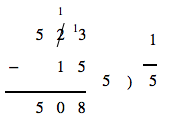The above describes the subtraction of 15 from 523, with a borrow from the ten's column to the one's column, as well as the division of 5 by 5 resulting in 1. Rendered using the MathML-mml3ff addon with Gecko as elementary math support is not natively available in Gecko.

##### Potential role mappings
• mstack = MATHML_STACK
• mlongdiv = MATHML_LONG_DIVISION
• msgroup = MATHML_STACK_GROUP
• msrow = MATHML_STACK_ROW
• mscarries = MATHML_STACK_CARRIES
• mscarry = MATHML_STACK_CARRY
• msline = MATHML_STACK_LINE

### Scripts and limits

These elements describe various scripts and limits. These include the following:

• msub - subscript, containing a base and a subscript
• msup - superscript, containing a base and a superscript
• msubsup - subscript and superscript, containing a base, a subscript, and a superscript
• munder - underscript, containing a base and an underscript
• mover - overscript, containing a base and an overscript
• munderover - underscript and overscript, containing a base, an underscript, and an overscript
• mmultiscripts - multiple scripts, with children described below

mmultiscripts uses a special syntax. The first child is the base, then any number of pairs of post-subscripts and post-superscripts. If prescripts are desired, a <mprescripts/> tag is included, then any number of pairs of pre-subscripts and pre-superscripts. <none/> can be substituted for scripts if no script is desired in a particular pair. Example markup is listed below.

<math display="block">
<msubsup>
<mi>b</mi>
<mn>1</mn>
<mn>2</mn>
</msubsup>
<mspace width="1em"/>
<munderover>
<mo>∑</mo>
<mrow>
<mi>n</mi>
<mo>=</mo>
<mn>1</mn>
</mrow>
<mn>5</mn>
</munderover>
<mi>n</mi>
<mo>=</mo>
<mn>15</mn>
<mspace width="1em"/>
<mmultiscripts>
<mi>x</mi>
<none/>
<mi>a</mi>
<mprescripts/>
<mi>b</mi>
<none/>
</mmultiscripts>
[/itex]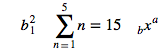The above describes the base b with subscript 1 and superscript 2, the summation of n from 1 to 5 being 15, and x with post-superscript a and pre-subscript b. Rendered using Gecko.

#### Potential role mappings

• msub = MATHML_SUB
• msup = MATHML_SUP
• msubsup = MATHML_SUB_SUP
• munder = MATHML_UNDER
• mover = MATHML_OVER
• munderover = MATHML_UNDER_OVER
• mmultiscripts = MATHML_MULTISCRIPTS

#### Potential relations

• underscript to parent structure (munder/munderover): MATHML_UNDER_OF
• parent structure to underscript: MATHML_UNDER
• overscript to parent structure (mover/munderover): MATHML_OVER_OF
• parent structure to overscript: MATHML_OVER
• base to parent structure (munder, mover, munderover, msub, msup, msubsup, mmultiscripts): MATHML_BASE_OF
• parent structure to base: MATHML_BASE
• subscript to parent structure (msub, msubsup): MATHML_SUBSCRIPT_OF
• parent structure to subscript: MATHML_SUBSCRIPT
• superscript to parent structure (msup, msubsup): MATHML_SUPERSCRIPT_OF
• parent structure to superscript: MATHML_SUPERSCRIPT
• pre-subscript to parent structure (mmultiscripts): MATHML_PRE_SUBSCRIPT_OF
• parent structure to pre-subscript: MATHML_PRE_SUBSCRIPT
• pre-superscript to parent structure (mmultiscripts): MATHML_PRE_SUPERSCRIPT_OF
• parent structure to pre-superscript: MATHML_PRE_SUPERSCRIPT
• post-subscript to parent structure (mmultiscripts): MATHML_POST_SUBSCRIPT_OF
• parent structure to post-subscript: MATHML_POST_SUBSCRIPT
• post-superscript to parent structure (mmultiscripts): MATHML_POST_SUPERSCRIPT_OF
• parent structure to post-superscript: MATHML_POST_SUPERSCRIPT

### Tables/matrices

These elements describe a table or matrix. This is also used for layout. These include the following:

• mtable - the container for a table, contains any number of mtr or mlabeledtr elements
• mtr - a row, containing any number of mtd elements
• mlabeledtr - a labelled row, having a caption on the side, containing any number of mtd elements
• mtd - a table cell, containing any number of elements

For mlabeledtr, the first mtd contained inside becomes the caption.

In addition, there are two elements used for table formatting:

• maligngroup - denotes a column in a table, used for alignment
• malignmark - denotes an alignment point inside an alignment group

malignmark is used inside a token element to set the alignment point. Example markup is listed below.

<mrow> <mo>[</mo> <mtable> <mtr> <mtd> <mn>1</mn> </mtd> <mtd> <mn>0</mn> </mtd> </mtr> <mtr> <mtd> <mn>0</mn> </mtd> <mtd> <mn>1</mn> </mtd> </mtr> </mtable> <mo>]</mo> </mrow> <mspace width="1em"/> <mtable> <mlabeledtr> <mtd> <mtext>Volume of a sphere</mtext> </mtd> <mtd> <mi>V</mi> <mo>=</mo> <mfrac> <mn>4</mn> <mn>3</mn> </mfrac> <mo>&InvisibleTimes;</mo> <mi>π</mi> <mo>&InvisibleTimes;</mo> <msup> <mi>r</mi> <mn>3</mn> </msup> </mtd> </mlabeledtr> </mtable> <mspace width="1em"/> <mtable> <mtr> <mrow> <mn>3</mn> <mo>&InvisibleTimes;</mo> <maligngroup/> <mi>x</mi> <maligngroup/> <mo>+</mo> <maligngroup/> <mi>y</mi> <maligngroup/> <mo>=</mo> <maligngroup/> <mn>7</mn> </mrow> </mtr> <mtr> <mrow> <mphantom> <mn>0</mn> <mo>&InvisibleTimes;</mo> <maligngroup/> <mi>x</mi> <maligngroup/> <mo>+</mo> </mphantom> <maligngroup/> <mi>y</mi> <maligngroup/> <mo>=</mo> <maligngroup/> <mn>1</mn> </mrow> </mtr> </mtable>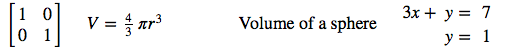The above describes the two-by-two identity matrix, the equation to determine the volume of a sphere, and two aligned equations. Rendered using MathJax due to lack of support of mlabeledtr, maligngroup, and malignmark in Gecko.

#### Potential role mappings

• mtable = MATHML_TABLE
• mtr = MATHML_LABELED_ROW
• mlabeledtr = MATHML_TABLE_ROW
• mtd = MATHML_CELL
• maligngroup = MATHML_ALIGNMENT_GROUP
• malignmark = MATHML_ALIGNMENT_MARK

### Semantics annotation

These elements provide explicit annotation for MathML. These include the following:

• semantics - starts a semantic annotation block, containing MathML and annotations
• annotation - annotation information in non-XML format
• annotation-xml - annotation information in XML format

The first child of semantics is the content being annotated, with the following annotation elements annotating the content.

Example markup is listed below.

$<semantics> <!-- Presentation MathML --> <mrow> <msup> <mi>x</mi> <mn>2</mn> </msup> <mo>+</mo> <mi>y</mi> </mrow> <!-- Content MathML --> <annotation-xml encoding="MathML-Content"> <apply> <plus/> <apply> <power/> <ci>x</ci> <cn type="integer">2</cn> </apply> <ci>y</ci> </apply> </annotation-xml> <!-- annotate TeX --> <annotation encoding="application/x-tex"> x^{2} + y </annotation> </semantics>$The above is the square of x added to y, with the content also annotated (invisibly) as Content MathML and TeX markup. Rendered using Gecko.

#### Potential role mappings

• semantics = MATHML_SEMANTICS
• annotation = MATHML_ANNOTATION
• annotation-xml = MATHML_XML_ANNOTATION

### Other

Other elements not fitting into the above categories.

• maction - binds actions to expressions
• merror - displays content as an error message

maction elements allow for interactivity with MathML content. Example markup is listed below.

$<merror> <mrow> <mtext>Division by zero: </mtext> <mfrac> <mn>1</mn> <mn>0</mn> </mfrac> </mrow> </merror>$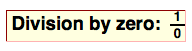The above is a divide by zero error. Rendered using Gecko.

$<maction actiontype="toggle"> <mfrac> <mn>6</mn> <mn>8</mn> </mfrac> <mfrac> <mrow> <mn>3</mn> <mo>⋅</mo> <mn>2</mn> </mrow> <mrow> <mn>4</mn> <mo>⋅</mo> <mn>2</mn> </mrow> </mfrac> <mfrac> <mn>3</mn> <mn>4</mn> </mfrac> </maction>$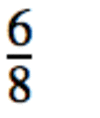The above is a togglable expression displaying 6/8, (3*2)/(4*2), and 3/4 upon each mouse press. Rendered using Gecko.

#### Potential role mappings

• maction = MATHML_ACTION
• merror = MATHML_ERROR

# Interface

## MathMLAccessible

Inherit from HyperTextAccessibleWrap.

• NativeAttributes - Override Accessible, and append MathML specific attributes if the element accepts them.
• RelationByType - Override Accessible, process MathML specific relations.

Attributes of interest might include the following:

• accent - Boolean value determining if the operator is an accent over the content.
• accentunder - Boolean value determining if the operator is an accent under the content.
• actiontype - String value specifying what an maction should do.
• align - String value specifying the type of alignment to be used for an mtable, munder, mover, munderover, or mstack. May be useful for determining logical ordering of an equation.
• bevelled - Boolean value determining rendering format of the fraction. May be useful for word choice (e.g. "one over two" vs. "one divided by two").
• close - String value for the closing delimiter of an mfenced element.
• columnlines - String value for the type of line between table columns. May be useful for determining table purpose.
• crossout - String value specifying the type of crossout to be used in an mscarry, changing the crossout's meaning.
• dir - String value (ltr, rtl) specifying text direction of the content.
• fence - Boolean value specifying whether an mo is a fence element.
• href - String value for hyperlinking to a URI.
• largeop - Boolean value for determining if an mo should be drawn larger. May be useful in determining semantics.
• linethickness - String or length value determining thickness of an mfrac line. May be useful in determining fraction's purpose (e.g. displaying binomial coefficients for a linethickness of 0).
• location - String (cardinal direction) value determining location of an mscarry relative to its anchoring element. May be useful in determining the carry's purpose.
• longdivstyle - String value controlling the appearance of an mlongdiv. May be useful in determining its usage.
• mathvariant - String value specifying the logical class of a token element. May be useful in determining a token's usage.
• notation - String value specifying the style to apply to the contents of an menclose.
• open - String value for the opening delimiter of an mfenced element.
• position - Integer value for mstack and mlongdiv child elements determining horizontal positioning.
• rowlines - String value for the type of line between table rows.
• selection - Integer value for determining which child element of an maction is to be used.
• separator - Boolean value specifying whether an mo is a separator.
• separators - String value containing separator values for an mfenced element.
• shift - Integer value for mstack and mlongdiv child elements determining vertical positioning.
• stretchy - Boolean value determining if an mo stretches to its adjacent elements' size. May be useful in determining operator usage.

MathML attributes will be added to/from this tentative list to be displayed under the accessible object attributes based on their usefulness to ATs.

Relations will link accessibles based on role. An example would be for a fraction structure, as follows.

$<mfrac> <mi>a</mi> <mn>2</mn> </mfrac>$


mfrac would have RELATION_NUMERATOR -> mi and RELATION_DENOMINATOR -> mn. mi would have RELATION_NUMERATOR_OF -> mfrac and mn would have RELATION_DENOMINATOR_OF -> mfrac.

A list of relations is as follows:

• MATHML_NUMERATOR_OF
• MATHML_NUMERATOR
• MATHML_DENOMINATOR_OF
• MATHML_DENOMINATOR
• MATHML_ROOT_INDEX_OF
• MATHML_ROOT_INDEX
• MATHML_UNDER_OF
• MATHML_UNDER
• MATHML_OVER_OF
• MATHML_OVER
• MATHML_BASE_OF
• MATHML_BASE
• MATHML_SUBSCRIPT_OF
• MATHML_SUBSCRIPT
• MATHML_SUPERSCRIPT_OF
• MATHML_SUPERSCRIPT
• MATHML_PRE_SUBSCRIPT_OF
• MATHML_PRE_SUBSCRIPT
• MATHML_PRE_SUPERSCRIPT_OF
• MATHML_PRE_SUPERSCRIPT
• MATHML_POST_SUBSCRIPT_OF
• MATHML_POST_SUBSCRIPT
• MATHML_POST_SUPERSCRIPT_OF
• MATHML_POST_SUPERSCRIPT
• MATHML_ROW_FOR
• MATHML_IN_ROW

## MathMLTableAccessible, MathMLTableRowAccessible, and MathMLTableCellAccessible

Inherit from HTMLTableAccessible, HTMLTableRowAccessible, and HTMLTableCellAccessible respectively.

Override NativeAttributes and RelationByType as with MathMLAccessible. The table elements can be traversed using the same interface as HTMLTableAccessible (nsIAccessibleTable and nsIAccessibleTableCell).

## Roles

One role for each of the following constructs (41 of them), plus the tag itself: #### Tokens • mi • mn • mo • mtext • mspace • ms • mglyph #### Layout (semantic meaning) • mrow • mfrac • msqrt • mroot • mfenced • menclose #### Layout (formatting) • mstyle • mpadded • mphantom #### Layout (elementary math) • mstack • mlongdiv • msgroup • msrow • mscarries • mscarry • msline #### Script/limits • msub • msup • msubsup • munder • mover • munderover • mmultiscripts #### Tables/matrices • mtable • mlabeledtr • mtr • mtd #### Table formatting • maligngroup • malignmark #### Semantics annotation • semantics • annotation • annotation-xml #### Other • maction • merror • math ### Sample Gecko roles • MATHML_MATH • MATHML_IDENTIFIER • MATHML_NUMBER • MATHML_OPERATOR • MATHML_TEXT • MATHML_SPACE • MATHML_STRING_LITERAL • MATHML_GLYPH • MATHML_ROW • MATHML_FRACTION • MATHML_SQUARE_ROOT • MATHML_ROOT • MATHML_FENCED • MATHML_ENCLOSED • MATHML_STYLE • MATHML_PADDED • MATHML_PHANTOM • MATHML_SUB • MATHML_SUP • MATHML_SUB_SUP • MATHML_UNDER • MATHML_OVER • MATHML_UNDER_OVER • MATHML_MULTISCRIPTS • MATHML_TABLE • MATHML_LABELED_ROW • MATHML_TABLE_ROW • MATHML_CELL • MATHML_ALIGNMENT_GROUP • MATHML_ALIGNMENT_MARK • MATHML_ACTION • MATHML_ERROR • MATHML_SEMANTICS • MATHML_ANNOTATION • MATHML_XML_ANNOTATION • MATHML_STACK • MATHML_LONG_DIVISION • MATHML_STACK_GROUP • MATHML_STACK_ROW • MATHML_STACK_CARRIES • MATHML_STACK_CARRY • MATHML_STACK_LINE ## Comparison with WebKit There's a decent mapping between the proposed interface and how WebKit currently implements MathML accessibility. As an example, the <msup> element will be used. [itex] <msup> <mi>x</mi> <mn>2</mn> </msup>


MathML elements are of AXRole AXGroup. In order to determine the type of MathML element, the AXSubrole is checked. The <msup> element has an AXSubrole of AXMathSubscriptSuperscript. In order to determine the type of MathML element it is (<msup>, <msub>, or <msubsup>), there are the following three attributes that are added:

• AXMathBase
• AXMathSubscript
• AXMathSuperscript

These attributes point to the element fulfilling each particular role, and have a nil value otherwise. In this case, since it's a superscript element, AXMathBase points to the <mi> and AXMathSuperscript points to the <mn>. AXMathSubscript has a nil value.

On the <mi> and <mn> elements, no additional information regarding MathML structure is given, apart from knowing that the sub-roles are AXMathIdentifier and AXMathNumber respectively. This is an advantage of the proposed interface, as it provides a way for child elements to know what part in their parent structure they are. <mi> would know it's the base, and <mn> would know it's the superscript in this case.

With regards to mapping the proposed interface back to a WebKit compatible format, the existing AccessibleWrap and RoleMap can be modified so that the same interface is provided on OS X. Additional information will also be available in the proposed interface (AXMathBaseOf or similar for the above example).